Function Repository Resource:

# OrthogonalPolynomialSum

Evaluate a finite orthogonal polynomial series

Contributed by: Jan Mangaldan
 ResourceFunction["OrthogonalPolynomialSum"][f,poly,x,{i,imin,imax}] evaluates the sum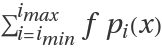, where pi(x) is an orthogonal polynomial represented by poly. ResourceFunction["OrthogonalPolynomialSum"][cof,poly,x] evaluates the sum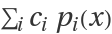, where ci is the (i+1)th element of the list cof.

## Details

The argument poly can be any of the following:
 "ChebyshevFirst" Chebyshev polynomial of the first kind ChebyshevT[i,x] "ChebyshevSecond" Chebyshev polynomial of the second kind ChebyshevU[i,x] "Hermite" Hermite polynomial HermiteH[i,x] "Laguerre" Laguerre polynomial LaguerreL[i,x] "Legendre" Legendre polynomial LegendreP[i,x] {"Gegenbauer",m} Gegenbauer polynomial GegenbauerC[i,m,x] {"Laguerre",a} associated Laguerre polynomial LaguerreL[i,a,x] {"Jacobi",a,b} Jacobi polynomial JacobiP[i,a,b,x]
Strings in specifications like "Legendre" and {"Jacobi",a,b} can be replaced with the corresponding symbols, as in LegendreP and {JacobiP,a,b}.
ResourceFunction["OrthogonalPolynomialSum"] uses Clenshaw summation to evaluate the sum.

## Examples

### Basic Examples (2)

Evaluate a finite Laguerre sum:

 In:=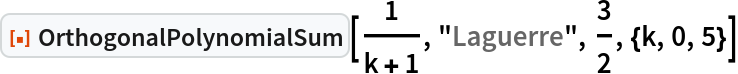Out=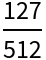Compare with the result of Sum:

 In:=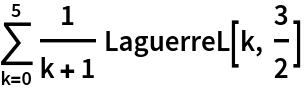Out=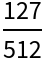### Scope (3)

Evaluate a finite Jacobi sum with symbolic coefficients and parameters:

 In:=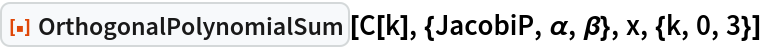Out=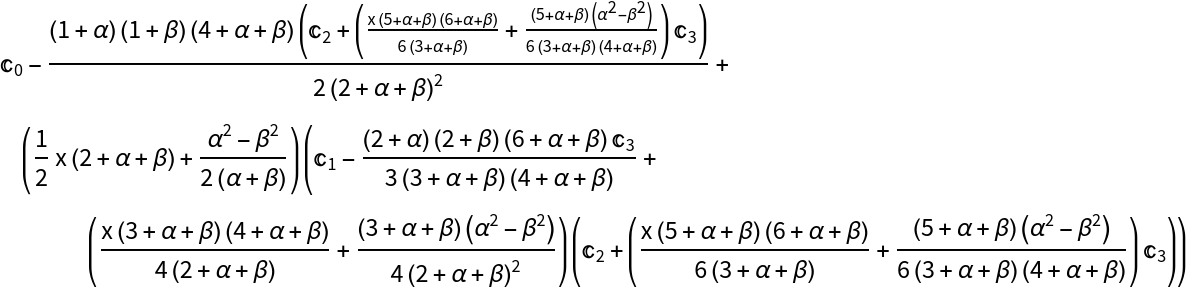Compare with an explicit summation:

 In:=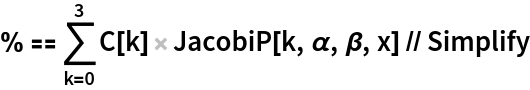Out=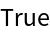Evaluate a partial sum of a Gegenbauer series:

 In:=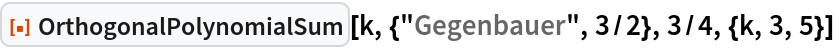Out=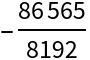Compare with an explicit summation:

 In:=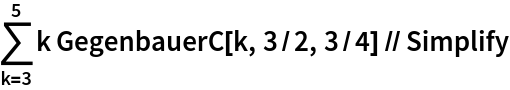Out=Evaluate a finite Hermite sum with coefficients supplied from a list:

 In:=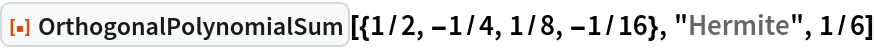Out=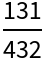Compare with an explicit evaluation:

 In:=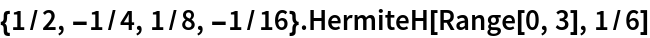Out=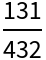### Properties and Relations (3)

Generate random coefficients of a Legendre series:

 In:=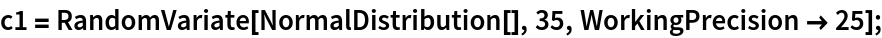Evaluating the Legendre series with OrthogonalPolynomialSum is faster than using LegendreP:

 In:=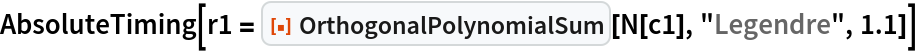Out=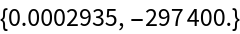In:=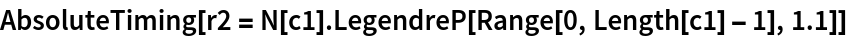Out=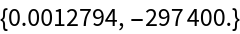The result obtained through OrthogonalPolynomialSum also has a slightly smaller error:

 In:=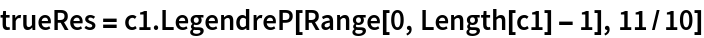Out=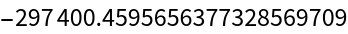In:=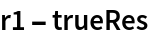Out=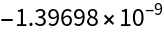In:=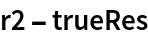Out=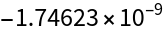## Version History

• 1.0.0 – 05 April 2021